# Brown-Douglas-Fillmore theory

(diff) ← Older revision | Latest revision (diff) | Newer revision → (diff)

BDF theory

The story of Brown–Douglas–Fillmore theory begins with the Weyl–von Neumann theorem, which, in one of its formulations, says that a bounded self-adjoint operator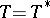on an infinite-dimensional separable Hilbert space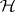is determined up to compact perturbations, modulo unitary equivalence, by its essential spectrum. (The essential spectrum is the spectrum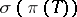of the imageofin the Calkin algebra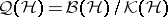; it is also the spectrum of the restriction ofto the orthogonal complement of the eigenspaces offor the eigenvalues of finite multiplicity; cf. also Spectrum of an operator.) In other words, unitary equivalence modulo the compacts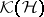washes out all information about the spectral measure of, and only the essential spectrum remains. This result was extended to normal operators (cf. also Normal operator) by I.D. Berg [a4] and W. Sikonia [a12], working independently. However, the theorem is not true for operators that are only essentially normal, in other words, for operatorssuch that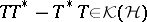. Indeed, the "unilateral shift"satisfiesand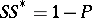, whereis a rank-one projection, yetcannot be a compact perturbation of a normal operator since its Fredholm index (cf. also Fredholm operator; Index of an operator) is non-zero. In [a2], L.G. Brown, R.G. Douglas and P.A. Fillmore (known to operator theorists as "BDF" ) showed that this is the only obstruction: an operatorin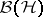is a compact perturbation of a normal operator if and only ifis essentially normal and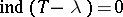for every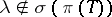.

However, they went considerably further, by putting this theorem in a-algebraic context in [a2] and [a3]. An operator"up to compact perturbations" defines an injective-homomorphism from a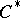-algebra(the closed subalgebra of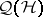generated by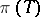and) to, and the-algebrais Abelian if and only ifis essentially normal. More generally, an extension of a separable-algebrais an injective-homomorphism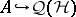, since this is equivalent to a commutative diagram with exact rows: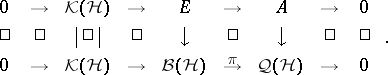BDF defined a natural equivalence relation (basically unitary equivalence) and an addition operation on such extensions, giving a commutative monoid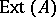, whose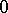-element is represented by split extensions (those for which there is a lifting). (The essential uniqueness of the split extensions was shown in [a14].) It was shown by M.D. Choi and E.G. Effros [a6] (see also [a1]) that this monoid is a group wheneveris nuclear (cf. also Nuclear space). (BDF originally worked only with Abelian-algebras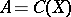, for which this is automatic, and they used the notation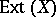for.) BDF showed that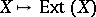behaves like a generalized homology theory in(cf. also Generalized cohomology theories), and in fact for finite CW-complexes (cf. also CW-complex) coincides with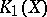, where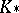is the homology theory dual to complex-theory. This was extended in [a7], where it was shown thatis canonically isomorphic to, Steenrod-homology (cf. also Steenrod–Sitnikov homology), for all compact metric spaces, and in [a5], where it was shown that on a suitable category of-algebras,fits into a short exact sequence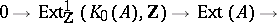It is now (as of 2000) known that BDF theory is just a special case of a more general theory of-algebra extensions. One type of generalization (see [a13]) involves replacingby the algebra of "compact" operators of a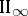factor (cf. also von Neumann algebra). Another sort of generalization involves replacingby an algebra of the form, whereis another separable (or-unital)-algebra. Theories of this sort were worked out in [a9], [a10] and in [a8], though the theory of [a9], [a10] turns out to be basically a special case of Kasparov's theory (see [a11]). Kasparov's-theory gives rise to a bivariant functor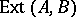, and whenis nuclear, this coincides [a8] with Kasparov's bivariant-functor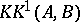.

How to Cite This Entry:
Brown-Douglas-Fillmore theory. Encyclopedia of Mathematics. URL: http://encyclopediaofmath.org/index.php?title=Brown-Douglas-Fillmore_theory&oldid=15203
This article was adapted from an original article by Jonathan Rosenberg (originator), which appeared in Encyclopedia of Mathematics - ISBN 1402006098. See original article